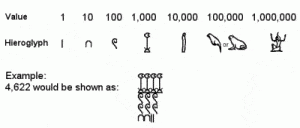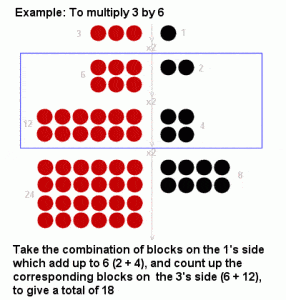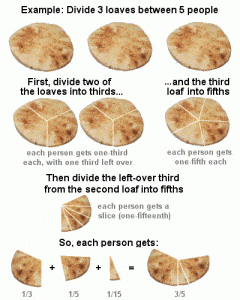# EGYPTIAN MATHEMATICS – NUMBERS & NUMERALSAncient Egyptian hieroglyphic numerals

The early Egyptians settled along the fertile Nile valley as early as about 6000 BCE, and they began to record the patterns of lunar phases and the seasons, both for agricultural and religious reasons.

The Pharaoh’s surveyors used measurements based on body parts (a palm was the width of the hand, a cubit the measurement from elbow to fingertips) to measure land and buildings very early in Egyptian history, and a decimal numeric system was developed based on our ten fingers. The oldest mathematical text from ancient Egypt discovered so far, though, is the Moscow Papyrus, which dates from the Egyptian Middle Kingdom around 2000 – 1800 BCE.

## Ancient Egyptian Number System

It is thought that the Egyptians introduced the earliest fully-developed base 10 numeration system at least as early as 2700 BCE (and probably much early). Written numbers used a stroke for units, a heel-bone symbol for tens, a coil of rope for hundreds and a lotus plant for thousands, as well as other hieroglyphic symbols for higher powers of ten up to a million. However, there was no concept of place value, so larger numbers were rather unwieldy (although a million required just one character, a million minus one required fifty-four characters).Ancient Egyptian method of multiplication

The Rhind Papyrus, dating from around 1650 BCE, is a kind of instruction manual in arithmetic and geometry, and it gives us explicit demonstrations of how multiplication and division was carried out at that time. It also contains evidence of other mathematical knowledge, including unit fractions, composite and prime numbers, arithmetic, geometric and harmonic means, and how to solve first order linear equations as well as arithmetic and geometric series. The Berlin Papyrus, which dates from around 1300 BCE, shows that ancient Egyptians could solve second-order algebraic (quadratic) equations.

Multiplication, for example, was achieved by a process of repeated doubling of the number to be multiplied on one side and of one on the other, essentially a kind of multiplication of binary factors similar to that used by modern computers (see the example at right). These corresponding blocks of counters could then be used as a kind of multiplication reference table: first, the combination of powers of two which add up to the number to be multiplied by was isolated, and then the corresponding blocks of counters on the other side yielded the answer. This effectively made use of the concept of binary numbers, over 3,000 years before Leibniz introduced it into the west, and many more years before the development of the computer was to fully explore its potential.

Practical problems of trade and the market led to the development of a notation for fractions. The papyri which have come down to us demonstrate the use of unit fractions based on the symbol of the Eye of Horus, where each part of the eye represented a different fraction, each half of the previous one (i.e. half, quarter, eighth, sixteenth, thirty-second, sixty-fourth), so that the total was one-sixty-fourth short of a whole, the first known example of a geometric series.Ancient Egyptian method of division

Unit fractions could also be used for simple division sums. For example, if they needed to divide 3 loaves among 5 people, they would first divide two of the loaves into thirds and the third loaf into fifths, then they would divide the left over third from the second loaf into five pieces. Thus, each person would receive one-third plus one-fifth plus one-fifteenth (which totals three-fifths, as we would expect).

The Egyptians approximated the area of a circle by using shapes whose area they did know. They observed that the area of a circle of diameter 9 units, for example, was very close to the area of a square with sides of 8 units, so that the area of circles of other diameters could be obtained by multiplying the diameter by 89 and then squaring it. This gives an effective approximation of π accurate to within less than one percent.

The pyramids themselves are another indication of the sophistication of Egyptian mathematics. Setting aside claims that the pyramids are first known structures to observe the golden ratio of 1 : 1.618 (which may have occurred for purely aesthetic, and not mathematical, reasons), there is certainly evidence that they knew the formula for the volume of a pyramid – 13 times the height times the length times the width – as well as of a truncated or clipped pyramid.

They were also aware, long before Pythagoras, of the rule that a triangle with sides 3, 4 and 5 units yields a perfect right angle, and Egyptian builders used ropes knotted at intervals of 3, 4 and 5 units in order to ensure exact right angles for their stonework (in fact, the 3-4-5 right triangle is often called “Egyptian”).